# Understanding Scale Development Process in Business Research

## Tutorial Focus

The tutorial focuses on answering two important questions

What is a Scale and Why need it?

What are the Key Steps in Scale Development?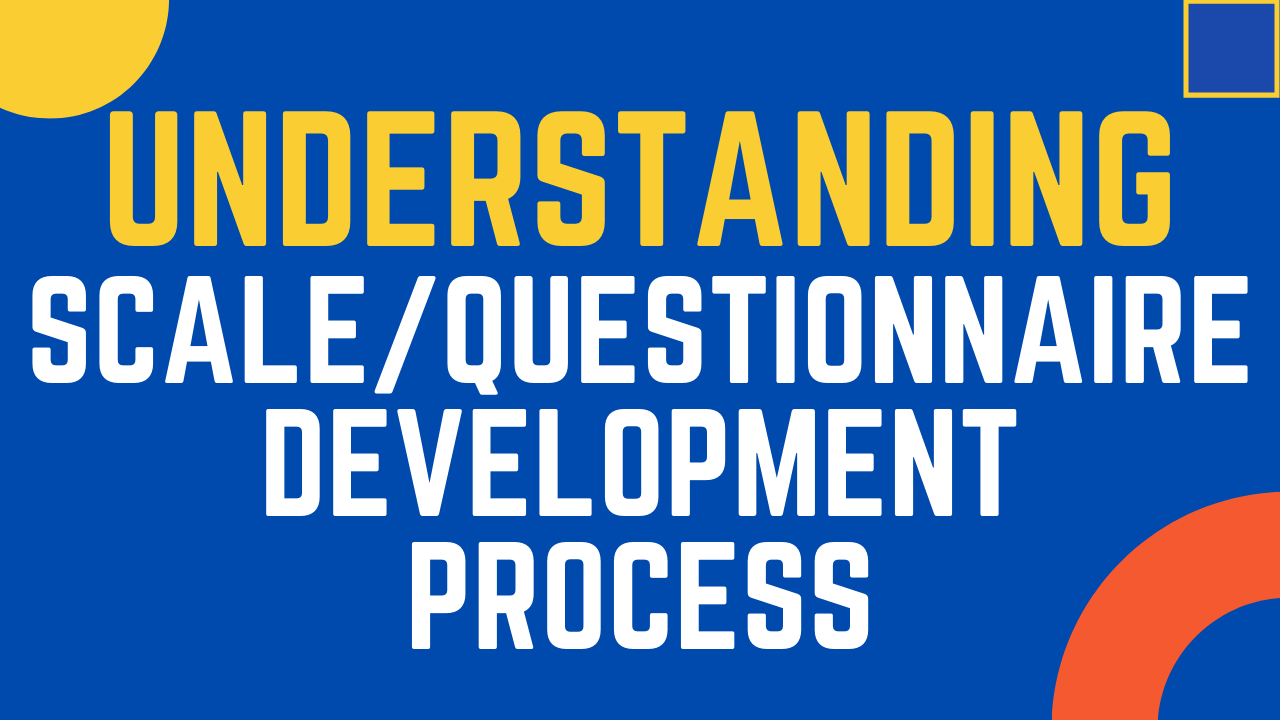### Understand Scale/Questionnaire Development Process

The tutorial discusses in detail the concept and steps involved in questionnaire/scale development process.

## What is a scale and why we need it

#### Questionnaire (also called a test or a scale) is defined as a set of items designed to measure one or more underlying constructs, also called latent variables (Fabrigar & Ebel-Lam, 2007).

In other words, it is a set of objective and standardized self-report questions whose responses are then summed up to yield a score. Item score is defined as the number assigned to performance on the item, task, or stimulus (Dorans, 2018: p. 578). Scale Development or construction, is the act of assembling or/and writing the most appropriate items that constitute test questions (Chadha, 2009) for a target population.

• The very first step is the operational definition of the constructs.
• Simply it means, How do you plan to operationalize (use) the construct in your study?
• How do you define the constructs in your study?
• The measurement is guided by the operational definition.

## Operational Definition

Operationalization is the process of strictly defining variables into measurable factors. The process defines vague concepts and allows them to be measured, empirically and quantitatively. Following are few example of operational definitions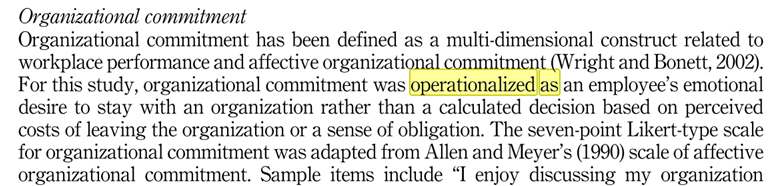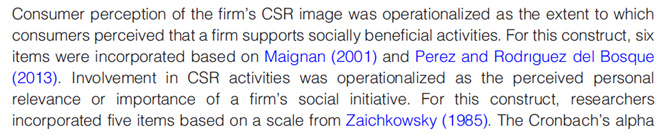## Scale Development Process

Following are the few most common  processes employed for scale development.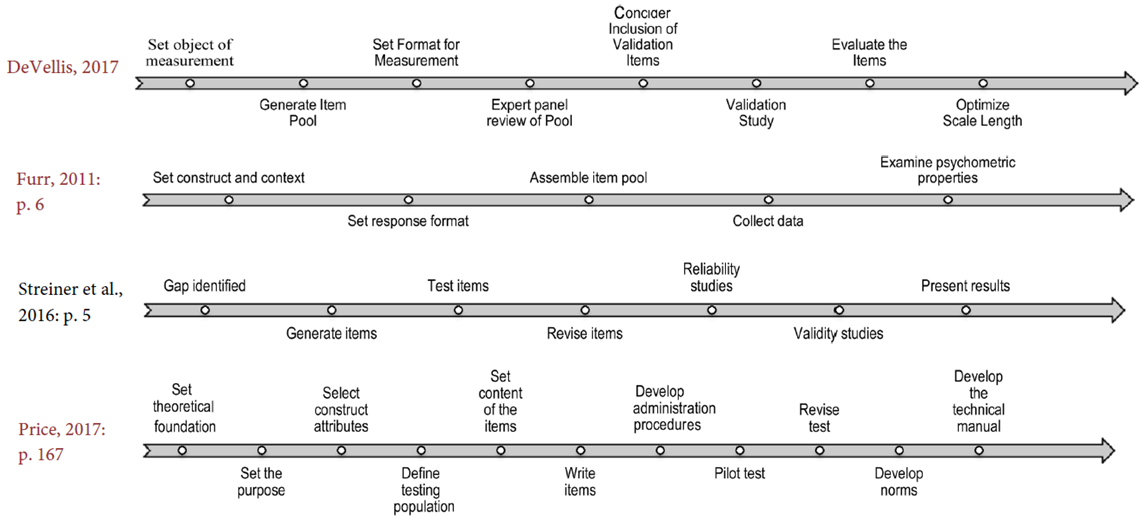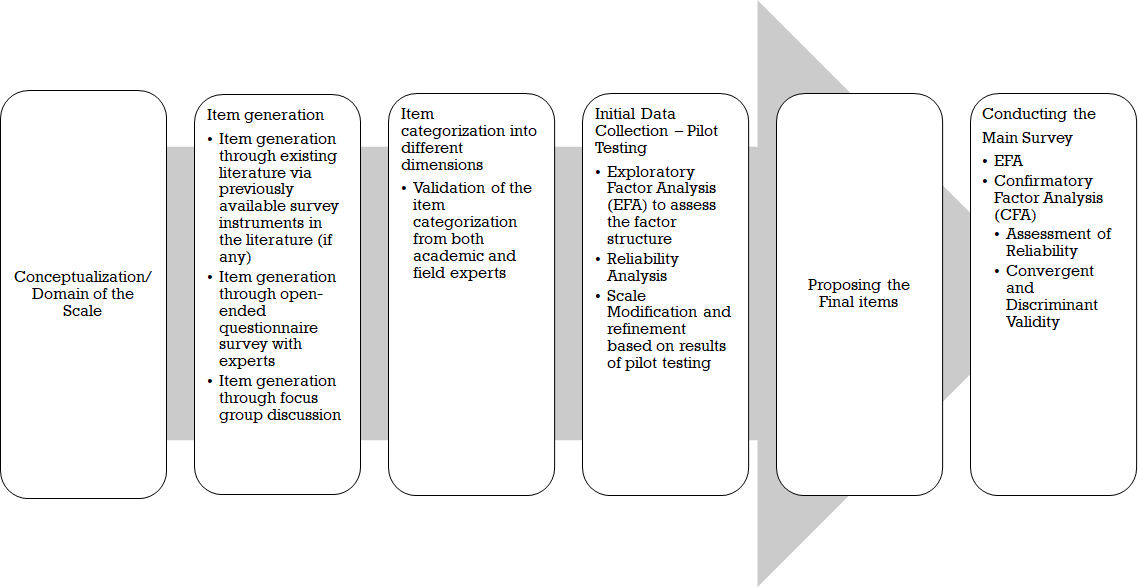## What is Factor Analysis?

• Factor analysis takes a large number of variables and reduces or summarizes it to represent them in different factors or components.
• Factor analysis is a method for investigating whether a number of variables of interest are linearly related to a smaller number of unobservable factors. This is done by grouping variables based on inter-correlations among set of variables.
• A common usage of factor analysis is in developing objective instruments for measuring constructs which are not directly observable in real life.
• Factor Analysis technique mainly examines the systematic interdependence among set of observed variables, and the researcher is mainly focused on determining the base of commonality among these variables.
• Factor analysis has been extensively used in research for data reduction and summarization. The main objective of factor analysis is to summarize the information contained in a large number of variables into a few small numbers of factors.
• How well do the items go well together. Incase we are building a new Scale.

## What is Measurement Model?

The measurement model is the part of the model that examines relationship between the latent variables and their measures. Measurement model helps establish the reliability and validity of the constructs